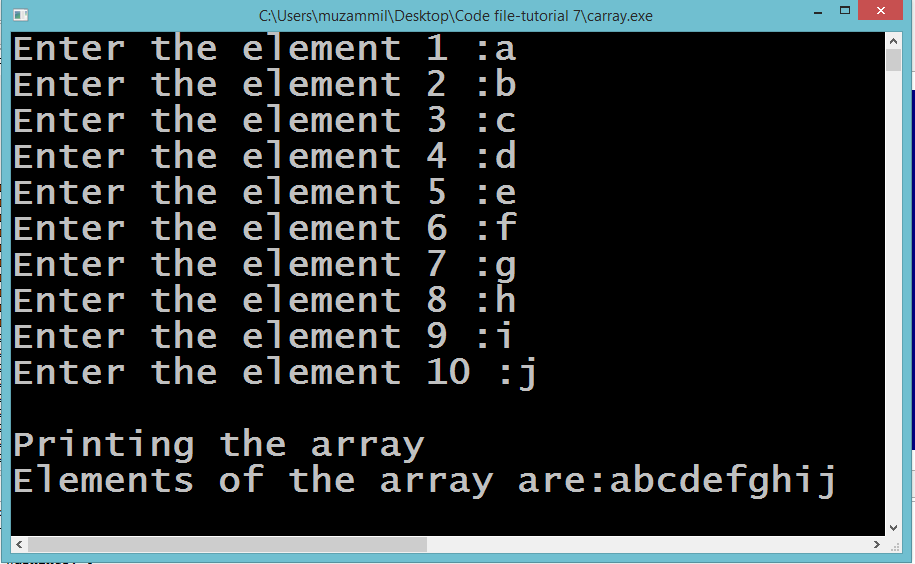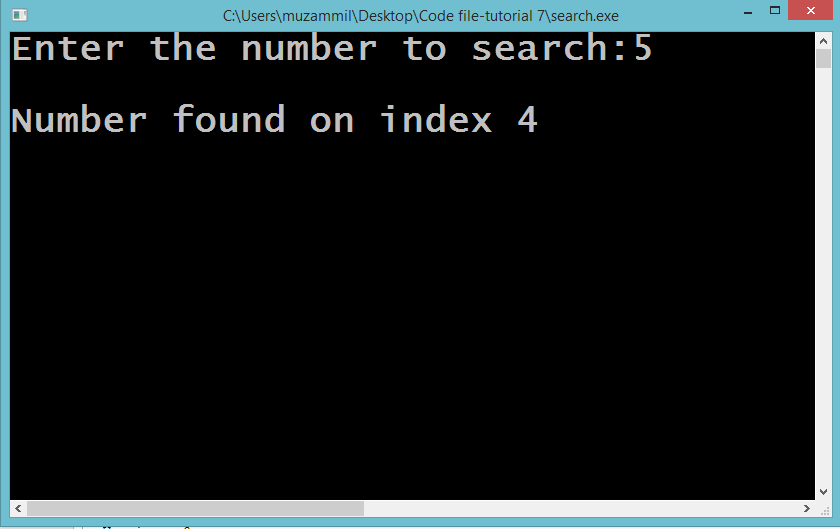# Introduction to Arrays

## Introduction to Arrays

In this part you will learn: 1. C syntax 2. Arrays 3. Showing output In this tutorial I will teach you about Arrays. Arrays are of extreme importance and use in C. Arrays are used when you want to create many variables of the same data type. Like if you want to note the grades of 100 students in a class you cannot take hundred character variables. It will be very lengthy and a not very good code. For this thing we use array. What we do is we just create an array of 100 spaces of character type. Like char array. Arrays Basic Step: Open Dev C++ then File > new > source file and start writing the code below.
`#include<stdio.h>#include<conio.h>`
These two are the most common and basic header files. The first one stdio.h is used to grant us most of the basic functions of C like Input and Output functions. The second one conio.h provides us functions like getch() which is used to pause our screen until we press a button.
`int main(){ 	char chararray; 	int i;	for( i=0;i<10;i++)	{		printf("Enter the element %d :",i+1);		scanf(" %c",&chararray[i]);	} 	printf("\nPrinting the array");	printf("\nElements of the array are:");	int j;	for( j=0;j<10;j++)	{		printf("%c",chararray[j]); 	}  	getch(); }`
In this program we created a character array of size 10. The number in the subscript following the name of variable is the size of array. This means your array will have 10 spaces. Which is same as having 10 character variables. Now after creating the array we took the input from the user in the array. This is done by writing a for loop and excessing the individual indexes of array one by one. You can see that in the scanf statement the place where we write the name of variable we wrote the name of our array and with that we place the subscript. This means we will take input in a specific index of the array. We ran the array 10 times because the size of our array is 10. And we want to take 10 inputs from the user. Now after taking the input its time to print all those elements that we just input in the array. So we wrote another for loop and ran it the same time as our size of the array. Then what we did is in the printf() statement where we write the name of the variable to print we wrote the name of the array with index in the subscript. What this will do is that it will print the value of only a specific element at a certain index. Execute > compile then Execute > run OutputSearching a number from the list Basic Step: Open Dev C++ then File > new > source file and start writing the code below.
`#include<stdio.h>#include<conio.h>`
These two are the most common and basic header files. The first one stdio.h is used to grant us most of the basic functions of C like Input and Output functions. The second one conio.h provides us functions like getch() which is used to pause our screen until we press a button.
`#include<stdio.h>#include<conio.h> int main(){ 	int list={1,2,3,4,5,6,7,8,9,10}; 	int s; 	printf("Enter the number to search:");	scanf("%d",&s);	bool found=false;	for(int i=0;i<10;i++)	{		if(s==list[i])		{			found=true;			printf("\nNumber found on index %d",i);			break;		}	}if(found==false)	{		printf("\nNumber not found in the list");	}  	getch(); }`
Here in this program we declared an integer array of size 10. We hardcoded 10 values in the array at the start. Then we took a number from the user which he wishes to find in the list of integers. Then we started a loop that checks our desired with every index of the list. If the number is found. The program print the index of the list where the number is found. Otherwise the program prints the number was not found in the list. Execute > compile then Execute > run Output